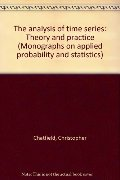# libib

## analysis of time series: Theory and practice (Monographs on applied probability and statistics), TheChristopher Chatfield
Time-series analysis is an area of statistics which is of particular interest at the present time. Time series arise in many different areas, ranging from marketing to oceanography, and the analysis of such series raises many problems of both a theoretical and practical nature. I first became interested in the subject as a postgraduate student at Imperial College, when I attended a stimulating course of lectures on time-series given by Dr. (now Professor) G. M. Jenkins. The subject has fascinated me ever since. Several books have been written on theoretical aspects of time-series analysis. The aim of this book is to provide an introduction to the subject which bridges the gap between theory and practice. The book has also been written to make what is rather a difficult subject as understandable as possible. Enough theory is given to introduce the concepts of time-series analysis and to make the book mathematically interesting. In addition, practical problems are considered so as to help the reader tackle the analysis of real data. The book assumes a knowledge of basic probability theory and elementary statistical inference (see Appendix III). The book can be used as a text for an undergraduate or postgraduate course in time-series, or it can be used for self tuition by research workers. Throughout the book, references are usually given to recent readily accessible books and journals rather than to the original attributive references. Wold's (1965) bibliography contains many time series references published before 1959.

No reviews
Item Posts
No posts